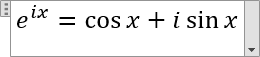# How to insert an equation with trigonometric functions

Word
This tip shows how to add an equation with trigonometric functions, for example, the Euler's formula.

How to add an equation in your document, see Working with Microsoft Equation.

To insert, for example, the Euler's formula, do the following:

## In the Professional format:

1.   In your own equation, on the Equation tab, in the Structures group, click the Script button:In the Script list, choose Superscript:2.   In the base box of the script, enter e.

3.   At the bottom of the script, enter ix.

4.   Enter =.

5.   On the Equation tab, in the Structures group, click the Function button:In the Function list, choose Cosine Function: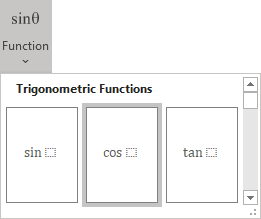6.   In the base box of the trigonometrical function, enter x.

7.   Enter +i.

8.   On the Equation tab, in the Structures group, click the Function button and then in the Function list, choose Sine Function: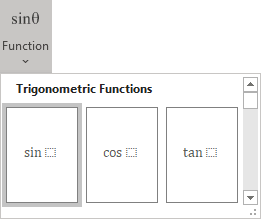9.   In the base box of the trigonometrical function, enter x: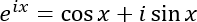## In the Linear format:

1.   In your own equation, enter e^(ix) and then press the space bar.

When you press a space key, this linear formula transformed to the professional format: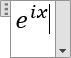2.   Enter =.

3.   Enter cos and then press the space bar: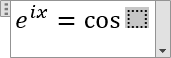4.   Enter x, then click the right arrow to leave the base box of the Cosine function.

5.   Enter +i and a space, then enter sin and a space again.

Then you press the space key, the second part of your linear formula transformed to the professional format: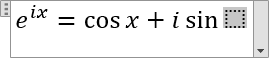6.   Enter x: